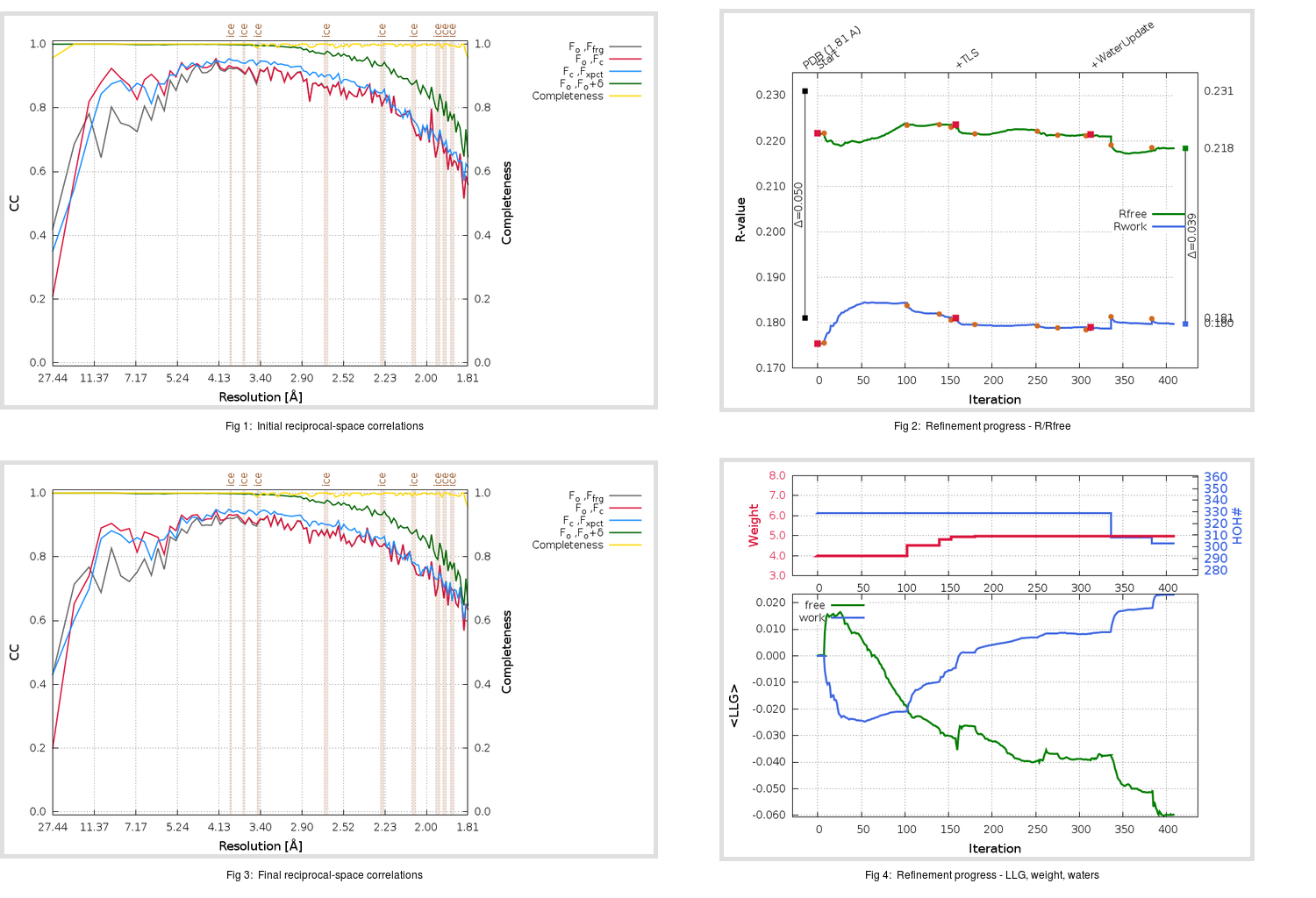Content:

```    Diffraction limits & principal axes of ellipsoid fitted to diffraction cut-off surface:
1.741         0.7458   0.0000   0.6662       0.969 a* + 0.249 c*
1.721         0.0000   1.0000   0.0000       b*
1.913        -0.6662   0.0000   0.7458      -0.887 a* + 0.462 c*
```

## Deposited

` `
 Date deposited Date data collection Resolution R, Rfree 20200315 20200304 1.81 0.1780 0.2310

Molprobity (CCP4 7.0 version) summary:

```Ramachandran outliers =   0.33 %
favored =  96.03 %
Rotamer outliers      =   0.38 %
C-beta deviations     =     0
Clashscore            =   4.63
RMS(bonds)            =   0.0132
RMS(angles)           =   1.85
MolProbity score      =   1.51
Resolution            =   1.81
R-work                =   0.1780
R-free                =   0.2310
```

```Number of waters      =   329

<B> (all atoms) =   26.26 ( sd =    9.60 ) for       2735 non-hydrogen atoms
<B>   (protein) =   24.80 ( sd =    8.77 ) for       2370 non-hydrogen atoms
<B>     (water) =   35.83 ( sd =    9.92 ) for        329 non-hydrogen atoms
<B>    (others) =   36.20 ( sd =   10.05 ) for         36 non-hydrogen atoms

B min/max       (all non-hydrogen atoms) =   13.76 /   82.94
B min/max   (protein non-hydrogen atoms) =   14.58 /   79.26
B min/max     (water non-hydrogen atoms) =   13.76 /   82.94
B min/max     (other non-hydrogen atoms) =   26.78 /   44.86
```

## BUSTER (re-)refinement

` `

Molprobity (CCP4 7.0 version) summary:

```Ramachandran outliers =   0.33 %
favored =  97.68 %
Rotamer outliers      =   1.14 %
C-beta deviations     =     0
Clashscore            =   2.95
RMS(bonds)            =   0.0115
RMS(angles)           =   1.59
MolProbity score      =   1.20
Resolution            =   1.81
R-work                =   0.1798
R-free                =   0.2184
```

```Number of waters      =   303

<B> (all atoms) =   26.80 ( sd =    8.87 ) for       2709 non-hydrogen atoms
<B>   (protein) =   25.23 ( sd =    7.44 ) for       2370 non-hydrogen atoms
<B>     (water) =   38.17 ( sd =   10.38 ) for        303 non-hydrogen atoms
<B>    (others) =   34.70 ( sd =   11.48 ) for         36 non-hydrogen atoms

B min/max       (all non-hydrogen atoms) =   11.80 /   78.76
B min/max   (protein non-hydrogen atoms) =   15.45 /   70.23
B min/max     (water non-hydrogen atoms) =   11.80 /   78.76
B min/max     (other non-hydrogen atoms) =   25.86 /   46.42
```

Refinement progression:Results:

` `
 File Remark 5REP_aB_refine.01_03_refine.pdb.gz exact refinement commands are in header 5REP_aB_refine.01_03_refine.mtz.gz including original deposited data and several re-refinement map coefficients 5REP_aB_refine.01_03_BUSTER_model.cif.gz including any non-standard compound restraints 5REP_aB_refine.01_03_BUSTER_refln.cif.gz